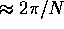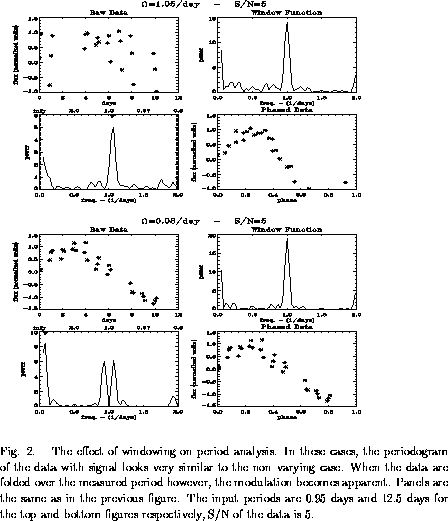Next: Simulations Up: Period Determination Previous: False Alarms and

## Windowing

The term ``spectral window'' is used to describe the response of the data analysis system to a perfect sine wave. If one observes a non-variable object with finite signal--to--noise each night at exactly the same time (equivalently an object with a one day period), the periodogram analysis on it will return a power spectrum that is peaked at 1 day. This means that there is a periodic signature from the sampling frequency itself. The observing times define a window. This window, convolved with the signal, determines the periodogram (Deeming 1975). The sampling frequency enters because you can define a period that is not the true period, yet hits all the observed points.

Figure 1 shows a typical analysis, including the window function. This demonstrates the main features of the window function. First there is a narrow central peak with widthwhere N equals the number of samples. The number of samples taken places a fundamental limit on the accuracy of the period measurement. Second, there are roughly evenly spaced side lobes. These side lobes contain spectral leakage due to high frequency components in the Fourier transform of the original signal. Third, the total power in the peak of the window function is greater than the power of the true signal by a factor of a few. Finally, there are large peaks on either side of the main lobe displace by one rotation per day. This is due to the one day periodicity in the observations. The windowing can cause a problem with period determination since periods very close to one day and very long periods and appear similar to the null signal case. Careful study of Figure 2 reveals difference between the window function and periodograms of these signals. Even with only 30 samples, periods deviating from a day by 1% are detectable. For convincing results though, the periodogram requires the aid of other data analysis. This usually consists of visual inspection of the raw data and the phased data. To gain a better understanding of how the observational data behave, simulations were run using synthetic data.Next: Simulations Up: Period Determination Previous: False Alarms and

Scott J. Wolk
Thu Dec 5 11:30:29 EST 1996